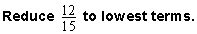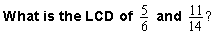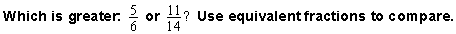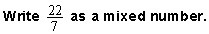# Practice Exercises: Fractions

Click once in an ANSWER BOX and type in your answer; then click ENTER. After you click ENTER, a message will appear in the RESULTS BOX to indicate whether your answer is correct or incorrect. To start over, click CLEAR.

• To enter the fraction three-fourths, type 3/4 into the form.
• To enter a mixed number, type the whole-number part and the fractional part, separated by a space. For example, to enter the mixed number four and two-thirds, type 4, a space, and then 2/3 into the form.
 1. Write a proper fraction using only the digits 8 and 3. ANSWER BOX:   RESULTS BOX:
 2. Write an improper fraction using only the digits 2 and 5. ANSWER BOX:   RESULTS BOX:
 3. Write the fraction five-sixths as an equivalent fraction with a denominator of 36. ANSWER BOX:   RESULTS BOX:
 4.ANSWER BOX:   RESULTS BOX:
 5.ANSWER BOX:   RESULTS BOX:
 6.ANSWER BOX:   RESULTS BOX:

#### Use the problem below for Exercises 7 and 8.

It takes Hope four-fifths of an hour to complete her social studies homework, three-fourths of an hour to complete her math homework, and five-sixths of an hour to complete her spelling homework. Order the time spent on Hope's homework by subject from least to greatest.

 7. Which subject took the least amount of time to complete? ANSWER BOX:   RESULTS BOX:
 8. Which subject took the most amount of time to complete? ANSWER BOX:   RESULTS BOX:
 9.ANSWER BOX:   RESULTS BOX:
 10. Write three and seven-eighths as an improper fraction. ANSWER BOX:   RESULTS BOX:
 Lessons on Fractions 1. Introduction to Fractions 2. Classifying Fractions 3. Equivalent Fractions 4. Simplifying Fractions 5. Comparing Fractions 6. Ordering Fractions 7. Converting Fractions to Mixed Numbers 8. Converting Mixed Numbers to Fractions 9. Practice Exercises 10. Challenge Exercises 11. Solutions